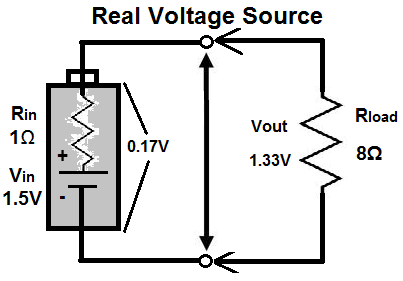﻿ Ideal Voltage Source Explained ﻿# Ideal Voltage Source ExplainedAn ideal voltage source is a voltage source that supplies constant voltage to a circuit despite the current which the circuit draws.

This means that despite the resistance which a load may be in a circuit, the source will still provide constant and steady voltage.

An ideal voltage source has the following characterstic that allows it to act as a 100% efficient source of voltage: it has zero internal resistance.

When an ideal voltage source has zero internal resistance, it can drop all of its voltage perfectly across a load in a circuit. Being that the source has zero internal resistance, none of the power is wasted due to internal resistance. The ideal voltage source can 100% efficiently drop all of its voltage across a load. This is proven by ohm's law. According to ohm's law, voltage is dropped across circuit elements according to the formula, V=IR. If a voltage source has zero internal resistance, it can drop all voltage across a load and none will be wasted internally. This is 100% power efficiency and this is an ideal voltage source.

Being that an ideal voltage source has zero internal resistance, and, thus, 100% efficiency to outputting all of its voltage to a load due to perfect voltage division, its voltage ouput to a load is steady and constant and doesn't fluctuate even if load resistance values change. Thus, the voltage output by an an ideal voltage source looks like:Let's examine the following circuit below:RIN, the internal resistance of the battery, is 0Ω. This demonstrates that this battery is an ideal voltage. The resistance load which is in the circuit is 8Ω. The load, therefore, receives all of the 1.5V of the battery.

This would be an ideal voltage source. However, this does not and cannot exist in real life, because all voltage sources, such as batteries, will have some type internal resistance. Due to voltage sources being physical objects, they will always have some degree of internal resistance which will make some of the voltage be wasted. Some have lower resistance than others, but all will have some resistance.

Real voltage sources, therefore, do have some resistance. This can vary from as little as a few thousandth of an ohm to as much as several ohms, such as 35Ω. Check out the article on Internal Resistance to learn more. This resistance makes the voltage source no longer act ideal. All of its voltage now will not be dropped across the load. Due to the internal resistance, the battery will drop some voltage across itself, leading to wasted power. The lower the resistance, the less wasted power.

Let's examine the same above circuit now with a voltage source with real conditions (some internal resistance):You see now how this circuit differs from the ideal one. Some power is wasted and there is not 100% efficiency. Because RIN is now 1Ω, there is no longer perfect conditions.

Although ideal voltage sources are impossible to make because all types have some internal resistance, the closer we can get a voltage source to ideal conditions, the more efficient the source will be in supplying voltage with little power waste. So we use ideal sources as models and try to make real components as close to them as possible.

Related Resources